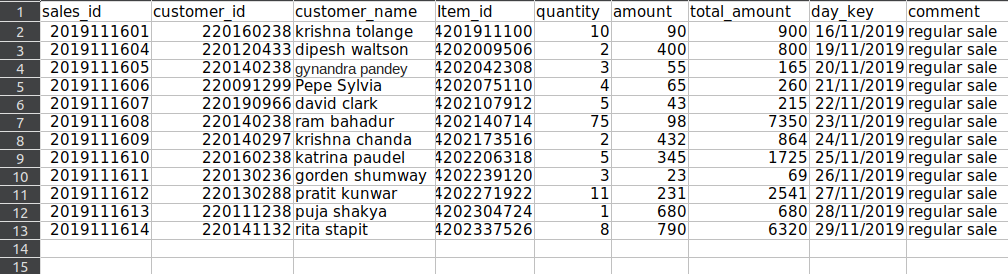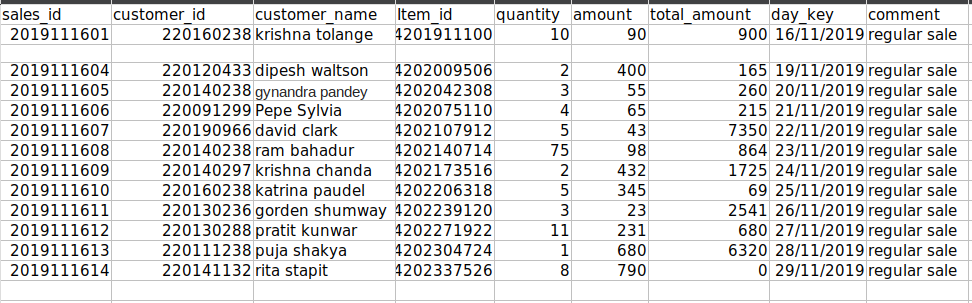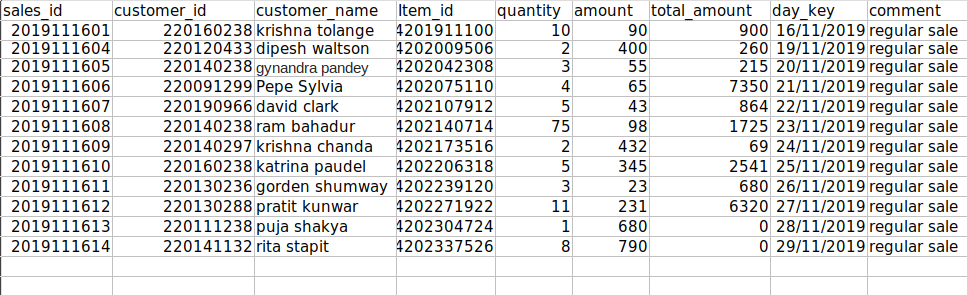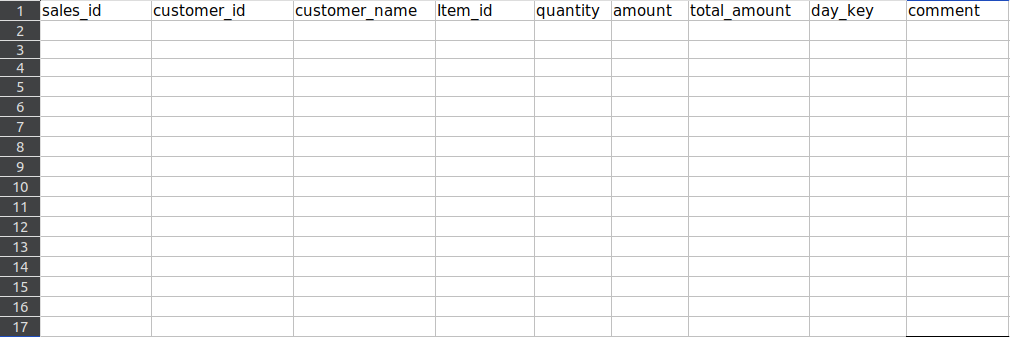# How to delete one or more rows in excel using Openpyxl?

• Last Updated : 03 Jun, 2022

Openpyxl is a Python library to manipulate xlsx/xlsm/xltx/xltm files. With Openpyxl you can create a new Excel file or a sheet and can also be used on an existing Excel file or sheet.

### Installation

This module does not come built-in with Python. To install this type the below command in the terminal.

`pip3 install openpyxl`

In this article, we will discuss how to delete rows in an Excel sheet with openpyxl. You can find the Excel file used for this article here.### Deleting Empty rows (one or more)

Method 1:

This method removes empty rows but not continues empty rows, because when you delete the first empty row the next row gets its position. So it is not validated. Hence, this problem can be solved by recursive function calls.

Approach:

• Import openpyxl library.
• Load Excel file with openpyxl.
• Then load the sheet from the file.
• Iterate the rows from the sheet that is loaded.
• Pass the row to remove the function.
• Then check for each cell if it is empty if any of the cells non-empty return the function, so only empty rows will exit the for loop without returning.
• Only if all rows are empty, remove statement is executed.
• Finally, save the file to the path.

## Python3

 `# import openpyxl library``import` `openpyxl` `# function to remove empty rows` `def` `remove(sheet, row):``    ``# iterate the row object``    ``for` `cell ``in` `row:``          ``# check the value of each cell in``        ``# the row, if any of the value is not``        ``# None return without removing the row``        ``if` `cell.value !``=` `None``:``              ``return``    ``# get the row number from the first cell``    ``# and remove the row``    ``sheet.delete_rows(row[``0``].row, ``1``)`  `if` `__name__ ``=``=` `'__main__'``:` `    ``# enter your file path``    ``path ``=` `'./delete_empty_rows.xlsx'` `    ``# load excel file``    ``book ``=` `openpyxl.load_workbook(path)` `    ``# select the sheet``    ``sheet ``=` `book[``'daily sales'``]` `    ``print``(``"Maximum rows before removing:"``, sheet.max_row)` `    ``# iterate the sheet object``    ``for` `row ``in` `sheet:``      ``remove(sheet,row)``       ` `    ``print``(``"Maximum rows after removing:"``,sheet.max_row)``    ` `    ``# save the file to the path``      ``path ``=` `'./openpy.xlsx'``    ``book.save(path)`

Output:

```Maximum rows before removing: 15
Maximum rows after removing: 14```

File after deletion:The first method deleted only the first empty row and the second continuous empty row is not deleted.

Method 2:

This method removes empty rows, including continuous empty rows by using the recursive approach. The key point is to pass the modified sheet object as an argument to the recursive function. If there is no empty row function is returned immediately.

Approach:

• Import openpyxl library.
• Load Excel file with openpyxl.
• Then load the sheet from the file.
• Pass the sheet that is loaded to the remove function.
• Iterate the rows with iter_rows().
• If any of the cells in a row is non-empty, any() return false, so it is returned immediately.
• If all cells in a row are empty, then remove the row with delete_rows().
• Then pass the modified sheet object to the remove function, this repeats until the end of the sheet is reached.
• Finally, save the file to the path.

## Python3

 `# import openpyxl library``import` `openpyxl` `# function to remove empty rows` `def` `remove(sheet):``  ``# iterate the sheet by rows``  ``for` `row ``in` `sheet.iter_rows():` `    ``# all() return False if all of the row value is None``    ``if` `not` `all``(cell.value ``for` `cell ``in` `row):` `      ``# detele the empty row``      ``sheet.delete_rows(row[``0``].row, ``1``)` `      ``# recursively call the remove() with modified sheet data``      ``remove(sheet)` `      ``return`  `if` `__name__ ``=``=` `'__main__'``:` `    ``# enter your file path``    ``path ``=` `'./delete_empty_rows.xlsx'` `    ``# load excel file``    ``book ``=` `openpyxl.load_workbook(path)` `    ``# select the sheet``    ``sheet ``=` `book[``'daily sales'``]` `    ``print``(``"Maximum rows before removing:"``, sheet.max_row)` `    ``# iterate the sheet``    ``for` `row ``in` `sheet:``      ``remove(sheet)``       ` `    ``print``(``"Maximum rows after removing:"``,sheet.max_row)` `    ` `    ``# save the file to the path``      ``path ``=` `'./openpy.xlsx'``    ``book.save(path)`

Output:

```Maximum rows before removing: 15
Maximum rows after removing: 13```

File after deletion:This method deleted both continuous empty rows as expected.

### Deleting All rows

Method 1:

In this method, we delete the second row repeatedly until a single row is left(column names).

Approach:

• Import openpyxl library.
• Load the Excel file and the sheet to work with.
• Pass the sheet object to delete function.
• Delete the second row, until there is a single row left.
• Finally, return the function.

## Python3

 `import` `openpyxl`  `def` `delete(sheet):` `    ``# continuously delete row 2 until there``    ``# is only a single row left over``    ``# that contains column names``    ``while``(sheet.max_row > ``1``):``        ``# this method removes the row 2``        ``sheet.delete_rows(``2``)``    ``# return to main function``    ``return`  `if` `__name__ ``=``=` `'__main__'``:` `        ``# enter your file path``    ``path ``=` `'./delete_every_rows.xlsx'` `    ``# load excel file``    ``book ``=` `openpyxl.load_workbook(path)` `    ``# select the sheet``    ``sheet ``=` `book[``'sheet1'``]` `    ``print``(``"Maximum rows before removing:"``, sheet.max_row)` `    ``delete(sheet)` `    ``print``(``"Maximum rows after removing:"``, sheet.max_row)` `    ``# save the file to the path``    ``path ``=` `'./openpy.xlsx'``    ``book.save(path)`

Output:

```Maximum rows before removing: 15
Maximum rows after removing: 1```

File after deletion:Method 2:

In this method, we use openpyxl sheet method to delete entire rows with a single command.

Approach:

• Import openpyxl library.
• Load the Excel file and the sheet to work with.
• Use delete_rows function to delete all rows except the column names.
• So a single empty row is left over.

## Python3

 `import` `openpyxl` `if` `__name__ ``=``=` `'__main__'``:` `    ``# enter your file path``    ``path ``=` `'./delete_every_rows.xlsx'` `    ``# load excel file``    ``book ``=` `openpyxl.load_workbook(path)` `    ``# select the sheet``    ``sheet ``=` `book[``'sheet1'``]` `    ``print``(``"Maximum rows before removing:"``, sheet.max_row)` `    ``# sheet.max_row is the maximum number``    ``# of rows that the sheet have``    ``# delete_row() method removes rows, first parameter represents row``    ``# number and sencond parameter represents number of rows``    ``# to delete from the row number``    ``sheet.delete_rows(``2``, sheet.max_row``-``1``)` `    ``print``(``"Maximum rows after removing:"``, sheet.max_row)` `    ``# save the file to the path``    ``path ``=` `'./openpy.xlsx'``    ``book.save(path)`

Output:

```Maximum rows before removing: 15
Maximum rows after removing: 1```

File after deletion:My Personal Notes arrow_drop_up